• 0 SARIMAX模型时间序列分析步骤 1.用pandas处理时序数据 2. 检验时序数据的平稳 3. 将时序数据平稳化 4. 确定order 的 p.d.q值 5. 确定season_order的四个值 6.应用SARIMAX模型对时序数据进行预测 其实...
0 SARIMAX模型时间序列分析步骤

1. 用pandas处理时序数据

2. 检验时序数据的平稳性

3. 将时序数据平稳化

4. 确定order 的 p.d.q值

5. 确定season_order的四个值

6. 应用SARIMAX模型对时序数据进行预测

其实SARIMAX比ARIMA模型就多了个season_order参数的确定，但也是这里最费时间的一个步骤

1 将数据转化成为时序数据

先一股脑导入一下工具包

import pandas as pd
import datetime
import matplotlib.pyplot as plt
from pylab import mpl
mpl.rcParams['font.sans-serif']=['SimHei']
import seaborn as sns
import statsmodels.tsa.stattools as ts
import statsmodels.api as sm
from statsmodels.tsa.arima_model import ARIMA
from statsmodels.stats.diagnostic import unitroot_adf
from statsmodels.graphics.tsaplots import plot_acf, plot_pacf
import itertools
import warnings
import numpy as np
from statsmodels.tsa.seasonal import seasonal_decompose

#读取数据
data = pd.read_csv('factor.csv')
data.index = pd.to_datetime(data['date'])
data.drop(['date'], axis=1, inplace=True)
data = data.result
data.head()

#数据大致情况展示
data.plot(figsize=(12,8))
plt.legend(bbox_to_anchor=(1.25, 0.5))
plt.title('result')
sns.despine()
plt.show()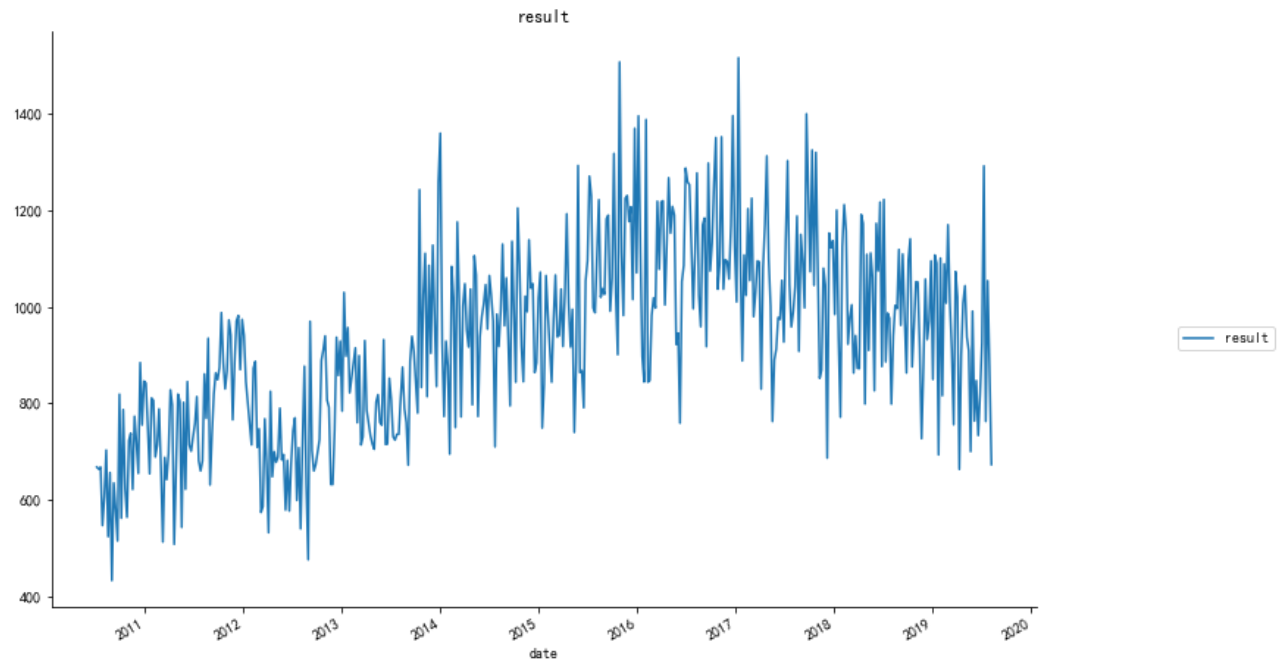2 序列平稳性检测

#数据平稳性检测 因为只有平稳数据才能做时间序列分析
def judge_stationarity(data_sanya_one):
dftest = ts.adfuller(data_sanya_one)
print(dftest)
dfoutput = pd.Series(dftest[0:4], index=['Test Statistic','p-value','#Lags Used','Number of Observations Used'])
stationarity = 1
for key, value in dftest.items():
dfoutput['Critical Value (%s)'%key] = value
if dftest > value:
stationarity = 0
print(dfoutput)
print("是否平稳(1/0): %d" %(stationarity))
return stationarity
stationarity = judge_stationarity(data)

3 序列平稳化

#若不平稳进行一阶差分
if stationarity == 0:
data_diff = data.diff()
data_diff = data_diff.dropna()
plt.figure()
plt.plot(data_diff)
plt.title('一阶差分')
plt.show()

#再次进行平稳性检测
stationarity = judge_stationarity(data_diff)

4 做一下季节性分解看看有没有季节性

#季节性分解
decomposition = seasonal_decompose(data,freq=28)
trend = decomposition.trend
seasonal = decomposition.seasonal
residual = decomposition.resid

plt.figure(figsize=[15, 7])
decomposition.plot()
print("test: p={}".format(ts.adfuller(seasonal)))

#季节平稳性检测
stationarity = judge_stationarity(residual)

5 对order参数p、q定阶，下面两种都可以，但图我还是看不来，下一种傻瓜式（稍微费时间）

#画ACF图和PACF图来确定p、q值
from statsmodels.graphics.tsaplots import plot_acf, plot_pacf

def draw_acf_pacf(ts,lags):
f = plt.figure(facecolor='white')
ax1 = f.add_subplot(211)
plot_acf(ts,ax=ax1,lags=lags)  #lags 表示滞后的阶数，值为30，显示30阶的图像
ax2 = f.add_subplot(212)
plot_pacf(ts,ax=ax2,lags=lags)
plt.subplots_adjust(hspace=0.5)
plt.show()
draw_acf_pacf(myts_diff,30)

#对模型p,q进行定阶
warnings.filterwarnings("ignore") # specify to ignore warning messages
from statsmodels.tsa.arima_model import ARIMA

pmax = int(5)    #一般阶数不超过 length /10
qmax = int(5)
bic_matrix = []
for p in range(pmax +1):
temp= []
for q in range(qmax+1):
try:
temp.append(ARIMA(data, (p, 1, q)).fit().bic)
except:
temp.append(None)
bic_matrix.append(temp)

bic_matrix = pd.DataFrame(bic_matrix)   #将其转换成Dataframe 数据结构
p,q = bic_matrix.stack().idxmin()   #先使用stack 展平， 然后使用 idxmin 找出最小值的位置
print(u'BIC 最小的p值 和 q 值：%s,%s' %(p,q))  #  BIC 最小的p值 和 q 值：0,1

6  通过网格搜索对seasonal_order进行定阶

#通过网格搜索对seasonal_order进行定阶,目前就是pdq=011，seasonal_order=2, 2, 1, 52效果比较好，RMSE=202.4582
def get_ARIMA_params(data, pdq, m=12):
p = d = q = range(0, 3)
seasonal_pdq = [(x, x, x, m) for x in list(itertools.product(p, d, q))]
score_aic = 1000000.0
warnings.filterwarnings("ignore") # specify to ignore warning messages
for param_seasonal in seasonal_pdq:
mod = sm.tsa.statespace.SARIMAX(data,
order=pdq,
seasonal_order=param_seasonal,
enforce_stationarity=False,
enforce_invertibility=False)
results = mod.fit()
print('x{}12 - AIC:{}'.format(param_seasonal, results.aic))
if results.aic < score_aic:
score_aic = results.aic
params = param_seasonal, results.aic
param_seasonal, results.aic = params
print('x{}12 - AIC:{}'.format(param_seasonal, results.aic))
pdq = [0, 1, 1]
get_ARIMA_params(data, pdq, m=52)

这上面最关键的是这个m值怎么设定，我设置默认为12。m是季节周期，参考别人代码的时候，月度数据的m为12，那我这里以周为单位，季节周期应该是52吧。一年12个月，52个星期，这个逻辑应该没有问题。

7  根据定阶参数进行模型拟合

mod = sm.tsa.statespace.SARIMAX(data,
order=(0, 1, 1),
seasonal_order=(2, 1, 2, 52),
enforce_stationarity=False,
enforce_invertibility=False)
results = mod.fit()
print(results.summary().tables)
results.plot_diagnostics(figsize=(15, 12))
plt.show()

这里模型拟合的时候用的数据的原始数据，而不是差分后的数据，因为order参数中已经设置了d为1，在拟合的时候会自动进行一阶差分，并在预测的时候对预测结果进行差分还原。

8  对预测值和真实值作图，并计算RMSE值作为评估参数

predict_ts = results.predict(tpy='levels')  #tpy='levels'直接预测值，没有的话预测的是差值
myts = data[predict_ts.index]  # 过滤没有预测的记录

predict_ts.plot(color='blue', label='Predict',figsize=(12,8))

myts.plot(color='red', label='Original',figsize=(12,8))

plt.legend(loc='best')
plt.title('RMSE: %.4f'% np.sqrt(sum((predict_ts-myts)**2)/myts.size))
plt.show()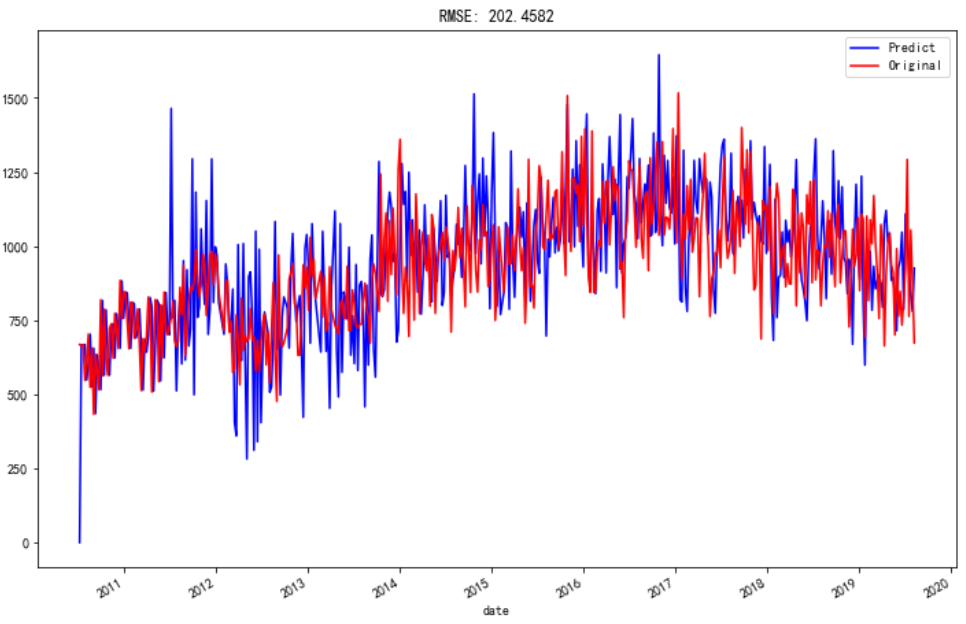9 向后对forecast值作图

steps = 20
start_time = myts.index[-1]
forecast_ts = results.forecast(steps)

fore = pd.DataFrame()
fore['date'] = pd.date_range(start=start_time ,periods=steps, freq='7D')
fore['result'] = pd.DataFrame(forecast_ts.values)
fore.index = pd.to_datetime(fore['date'])

predict_ts['2019/1/18':].plot(color='blue', label='Predict',figsize=(12,8))
myts['2019/1/18':].plot(color='red', label='Original',figsize=(12,8))
fore.result.plot(color='black', label='forecast',figsize=(12,8))

plt.legend(loc='best')
plt.show()

这里有个函数pd.date_range是专门用于产生时间序列索引的，start = 开始时间，end = 结束时间，periods=时间索引的个数，freq=‘7D’表示7天为一个时间索引间隔，也可以是'7W'七周，'M'一个月等等。

由于预测的数据没有时间索引，只有序号所以我要在这给他生成时间索引，并合并到dataframe，这样就可以和其他值一起在图像上展示了。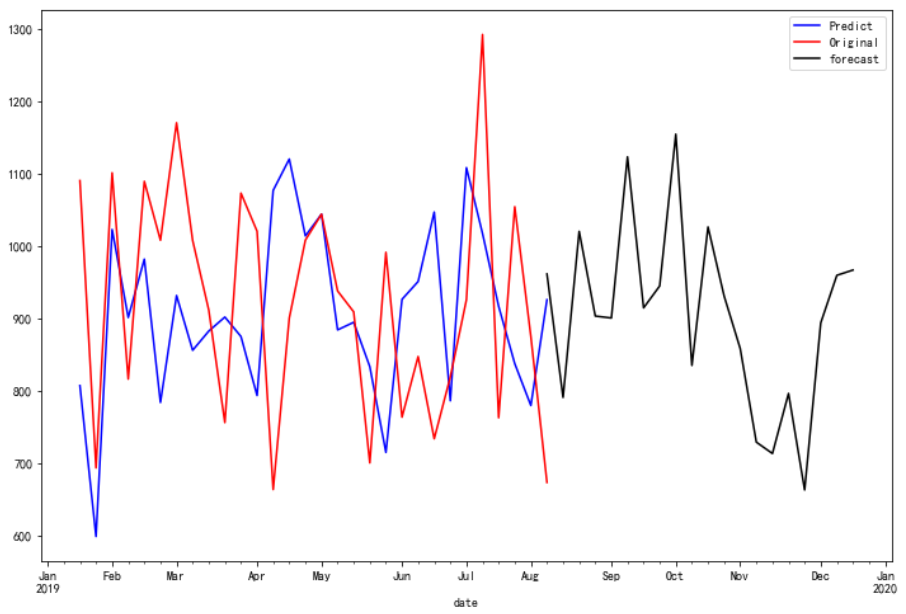最后forecast的效果还是可以的嘛，保存forecast文件

fore.to_csv('forecast_20steps.csv')


展开全文ARIMA
• 在我们的研究中，我们考虑了对印度旁遮普省降雨数据进行统计分析的季节性和周期性时间序列模型。 在本研究论文中，我们应用季节性自回归综合移动平均和周期自回归模型来分析旁遮普省的降雨数据。 为了评估模型识别...
• 1.季节性时间序列 包含：长期趋势Trend，季节趋势Seasonal，周期循环Circle，随机项Random 这里分解为相加模型X=T+S+C+R   在对时间序列进行分解之前，应该对序列进行检验：（下次写） 2.decompose()函数 将...
1.季节性时间序列

包含：长期趋势Trend，季节趋势Seasonal，周期循环Circle，随机项Random

这里分解为相加模型X=T+S+C+R

在对时间序列进行分解之前，应该对序列进行检验：（下次写）

2.decompose()函数

将时间序列进行上述分解

3.R分解操作过程

3.1数据读入与可视化

>#以纽约市月出生数量（1946.1-1959.12）的数据集为例

> births <-scan("http://robjhyndman.com/tsdldata/data/nybirths.dat")

Read 168 items

> birthstimeseries <- ts(births, frequency=12, start=c(1946,1))

> plot(birthstimeseries)出生数量

从图上可以看出，出生数量具有一定的季节性（夏峰冬谷）和周期性，同时趋势性明显；但是每个周期内的波动幅度变化较小，且不随时间趋势而变化，随便波动项随时间变化页不明显。

3.时间序列分解

分解为加法模型

>birthcomponents <- decompose(birthstimeseries)

> plot(birthcomponents)分解图

4.剔除季节因素

可以对季节性等进行剔除，现剔除季节因素

>birthstimeseriesseasonallyadjusted<-birthstimeseries-birthcomponents\$seasonal

>plot(birthstimeseriesseasonallyadjusted)出生数量（剔除季节因素）
展开全文• ## 算法模型---时间序列模型

万次阅读 多人点赞 2018-01-16 09:04:58
1、时间序列时间序列是时间间隔不变的情况下收集的不同时间点数据集合，这些集合被分析用来了解长期发展趋势及为了预测未来。...常用的时间序列模型有AR模型、MA模型、ARMA模型和ARIMA模型等。2、时间
文章来源
时间序列
时间序列是时间间隔不变的情况下收集的不同时间点数据集合，这些集合被分析用来了解长期发展趋势及为了预测未来。
时间序列与常见的回归问题的不同点在于:
1、时间序列是跟时间有关的;而线性回归模型的假设：观察结果是独立的在这种情况下是不成立的。
2、随着上升或者下降的趋势，更多的时间序列出现季节性趋势的形式；
常用的时间序列模型有AR模型(Autoregressive model:自回归模型)、MA模型(moving average model:滑动平均模型)、ARMA模型(Auto-Regressive and Moving Average Model:自回归滑动平均模型)和ARIMA模型(Autoregressive Integrated Moving Average Model:自回归积分滑动平均模型)等。
时间序列的预处理(使数据平稳化和随机化)
拿到一个观察值序列之后，首先要对它的平稳性和纯随机性进行检验，这两个重要的检验称为序列的预处理。根据检验的结果可以将序列分为不同的类型，对不同类型的序列我们会采用不同的分析方法。
平稳化处理
平稳 就是围绕着一个常数上下波动且波动范围有限，即有常数均值和常数方差。如果有明显的趋势或周期性，那它通常不是平稳序列。序列平稳不平稳，一般采用三种方法检验：
时序图检验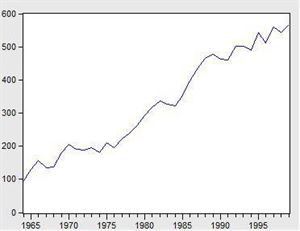看看上面这个图，很明显的增长趋势，不平稳。
利用自相关系数和偏相关系数
自相关系数和偏相关系数的概念可参考《算法模型— 概率论基础—相关系数相关》
下面是不平稳数据的自相关和偏相关系数的一种情形。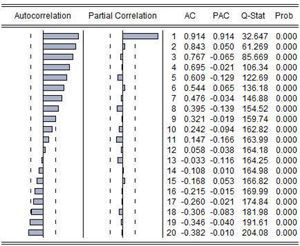左边第一个为自相关图（Autocorrelation），第二个偏相关图(Partial Correlation)。
平稳的序列的自相关图和偏相关图要么拖尾，要么是截尾。截尾就是在某阶之后，系数都为 0 。怎么理解呢，看上面偏相关的图，当阶数为 1 的时候，系数值还是很大， 0.914;二阶长的时候突然就变成了 0.050. 后面的值都很小，认为是趋于 0 ，这种状况就是截尾。什么是拖尾，拖尾就是有一个缓慢衰减的趋势，但是不都为 0 。
自相关图既不是拖尾也不是截尾。以上的图的自相关是一个三角对称的形式，这种趋势是单调趋势的典型图形，说明这个序列不是平稳序列。
平稳序列的自相关系数会快速衰减。
单位根检验
单位根检验是指检验序列中是否存在单位根，如果存在单位根就是非平稳时间序列。
单位根检验：ADF是一种常用的单位根检验方法，他的原假设为序列具有单位根，即非平稳，对于一个平稳的时序数据，就需要在给定的置信水平上显著，拒绝原假设。ADF只是单位根检验的方法之一，如果想采用其他检验方法，可以安装第三方包arch，里面提供了更加全面的单位根检验方法，个人还是比较钟情ADF检验。以下为检验结果，其p值大于0.99，说明并不能拒绝原假设。
利用差分将序列数据转换为平衡序列
差分可以将数据转换为平稳序列。
一阶差分指原序列值相距一期的两个序列值之间的减法运算；k阶差分就是相距k期的两个序列值之间相减。如果一个时间序列经过差分运算后具有平稳性，则该序列为差分平稳序列，可以使用ARIMA模型进行分析。
确定不平稳后，依次进行1阶、2阶、3阶…差分，直到平稳为止。
随机化处理
对于纯随机序列，又称白噪声序列，序列的各项数值之间没有任何相关关系，序列在进行完全无序的随机波动，可以终止对该序列的分析。白噪声序列是没有信息可提取的平稳序列。对于平稳非白噪声序列，它的均值和方差是常数。通常是建立一个线性模型来拟合该序的发展，借此提取该序列的有用信息。ARMA模型是最常用的平稳序列拟合模型。
平稳时间序列建模
某个时间序列经过预处理，被判定为平稳非白噪声序列，就可以进行时间序列建模。
建模步骤：
（1）计算出该序列的自相关系数（ACF）和偏相关系数（PACF）；
（2）模型识别，也称模型定阶。根据系数情况从AR§模型、MA(q)模型、ARMA(p，q)模型、ARIMA（p，d，q）模型中选择合适模型，其中p为自回归项，d为差分阶数，q为移动平均项数。
若平稳序列的偏相关函数是截尾的，而自相关函数是拖尾的，可断定序列适合AR模型；若平稳序列的偏相关函数是拖尾的，而自相关函数是截尾的，则可断定序列适合MA模型；若平稳序列的偏相关函数和自相关函数均是拖尾的，则序列适合ARMA模型。（截尾是指时间序列的自相关函数（ACF）或偏自相关函数（PACF）在某阶后均为0的性质（比如AR的PACF）；拖尾是ACF或PACF并不在某阶后均为0的性质（比如AR的ACF）。）
（3）估计模型中的未知参数的值并对参数进行检验；
（4）模型检验；
（5）模型优化；
（6）模型应用：进行短期预测。
混合自回归移动平均过程（公式表达）
差分方程
所谓差分方程即将变量 $y_t$与它的滞后期联系起来的表达式。
研究变量在第t期的值记为$y_t$。假定给出的动态方程将变量y第t期的值与另外的变量$w_t$以及y的前一期联系起来:
$y_t=ϕy_{t−1}+w_t$
上述称为一阶差分方程是因为仅仅只有变量的一阶滞后$(y_t−1)$出现在方程中。
移动平均
“移动平均”的含义源于$Y_t$是最近两期的$\epsilon$的加权平均。
令${\epsilon_t}$是一个白噪声序列。
$Y_t=μ+\epsilon_t+θ\epsilon_{t−1}$
其中μ和θ可以是任意的常数。这个时间序列称为一阶移动平均过程，记为$MA(1)$。
自回归过程
一阶自回归过程
一个一阶自回归，记作$AR(1)$满足下面的差分方程
$Y_t=c+ϕY_{t−1}+\epsilon_t$
${\epsilon_t}$是一个白噪声序列。
p阶自回归过程
一个p阶自回归，记作$AR(p)$满足下式
$Y_t=c+ϕ_1Y_{t−1}+ϕ_2Y_{t−2}+...+ϕ_pY{t−p}+\epsilon_t$
混合自回归移动平均过程
一个$ARMA(p,q)$过程包括自回归和移动平均项：
$Y_t=c+ϕ_1Y_{t−1}+ϕ_2Y_{t−2}+...+ϕ_pY{t−p}+\epsilon_t+θ_1ϵ_{t−1}+θ_2ϵ{t−2}+...+θ_qϵ_{t−q}$
利用statsmodels库实现时间序列的分析处理
Time Series analysis tsa
statsmodels.tsa.arima_model.ARIMA
AR(I)MA时间序列建模过程——步骤和python代码
Seasonal ARIMA with Python
Python 3中使用ARIMA进行时间序列预测的指南
Python_Statsmodels包_时间序列分析_ARIMA模型
时间序列实战（一）
How to Make Predictions for Time Series Forecasting with Python
How to Use and Remove Trend Information from Time Series Data in Python
时间序列分析之ARIMA上手-Python
[python] 时间序列分析之ARIMA
python中有专门的库statsmodels可以用来作时间序列分析，但spark中则没有现成的。
#-*- coding: utf-8 -*-
import matplotlib.pyplot as plt
import pandas as pd
data=pd.read_csv('./HIS_MONTH.csv')
b=data['fData']
plt.plot(data['fData'])
plt.show()

数据结构如下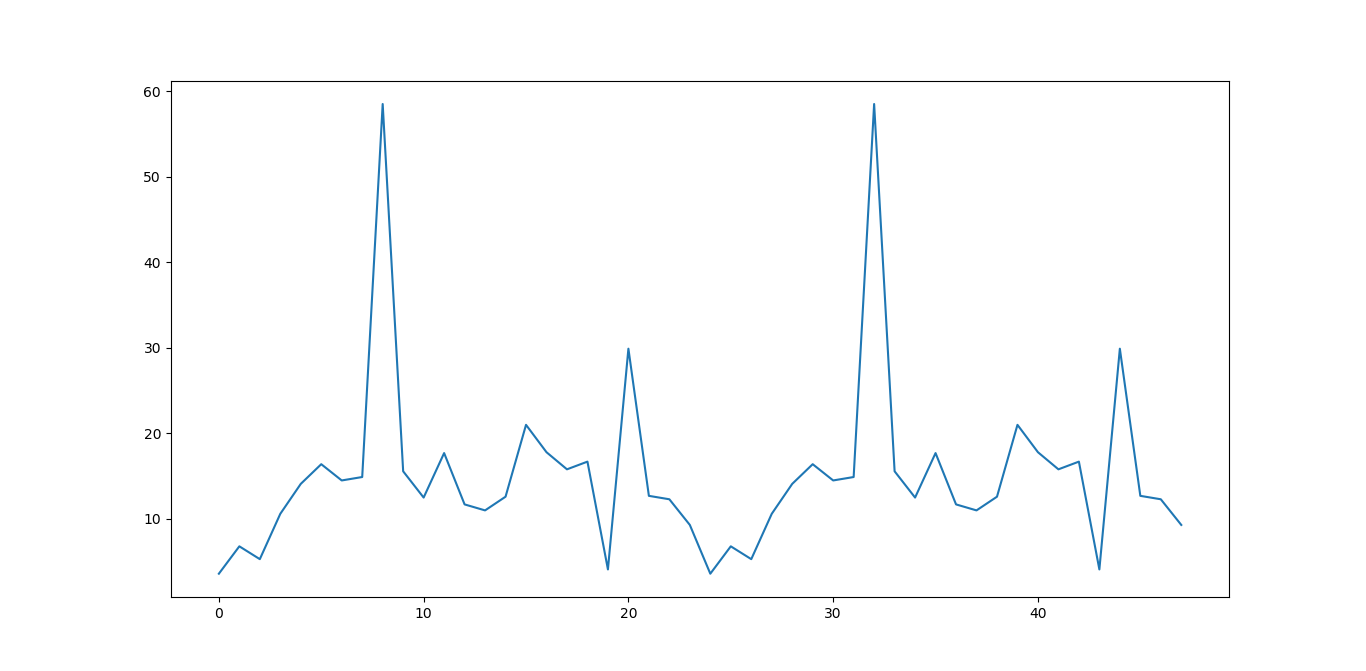#自相关图
from statsmodels.graphics.tsaplots import plot_acf
plot_acf(data['fData']).show()

如下所示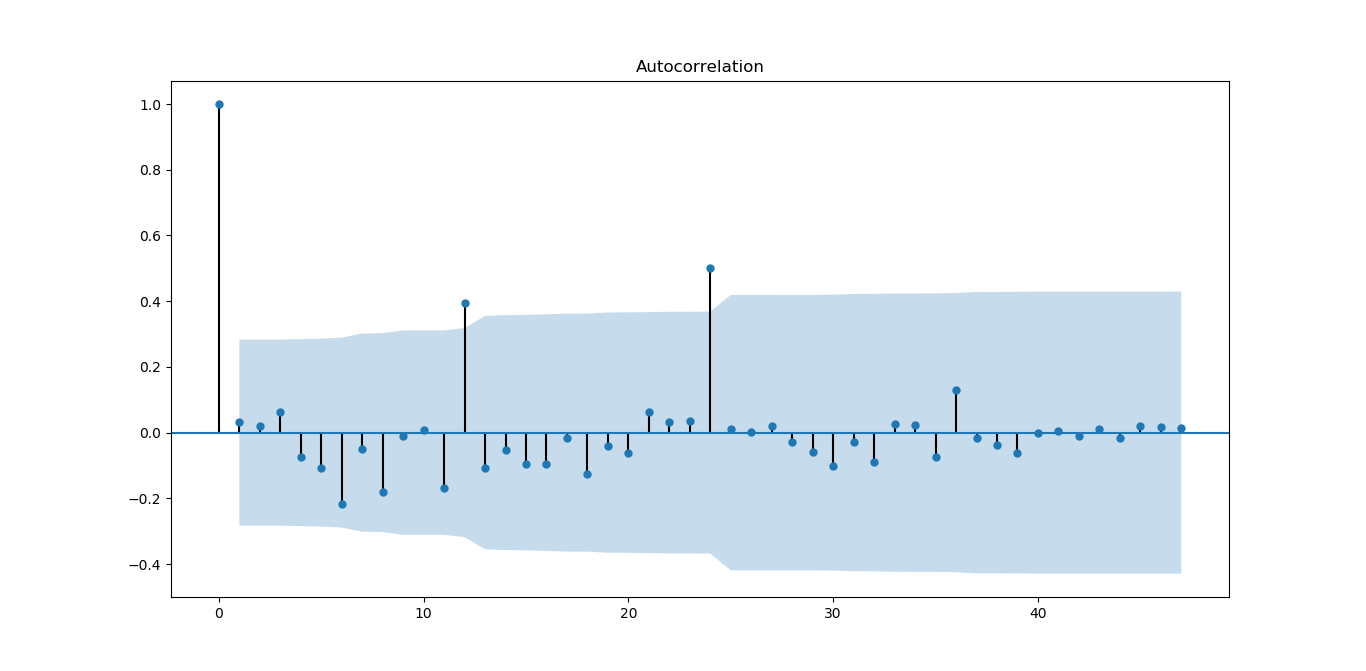从图上可以自相关系数基本是拖尾的，稍做调整就可以使用。
#偏自相关图
from statsmodels.graphics.tsaplots import plot_pacf
plot_pacf(data['fData']).show()

如下图所示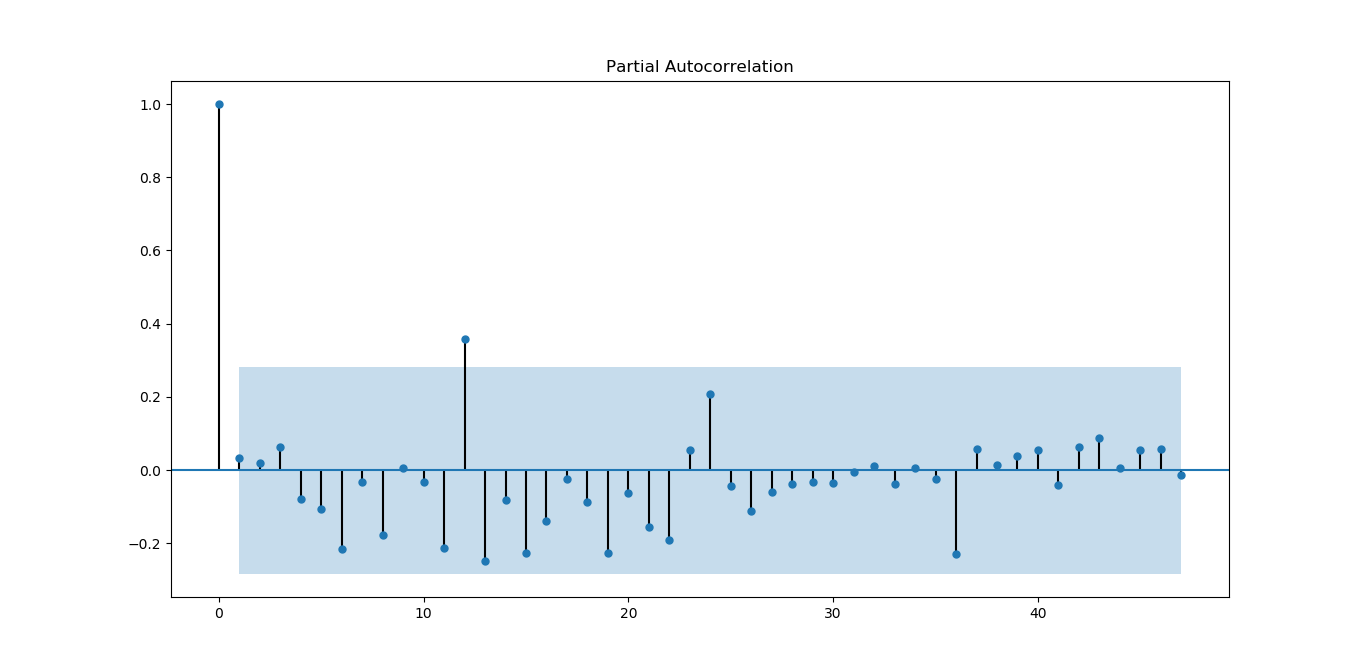偏相关系数也基本符合拖尾。
#平稳性检测
from statsmodels.tsa.stattools import adfuller as ADF
print('原始序列的ADF检验结果为：')
print(ADF(data['fData']))
#返回值依次为adf、pvalue、usedlag、nobs、critical values、icbest、regresults、resstore

如下所示
原始序列的ADF检验结果为：
(-6.561077625309946, 8.378411469638636e-09, 0L, 47L, {'5%': -2.925338105429433, '1%': -3.5778480370438146, '10%': -2.6007735310095064}, 273.5266547319719)

p值远小于0.05，拒绝原假设（原假设认为存在单位根），所以该序列为平衡时间序列。
#白噪声检验
from statsmodels.stats.diagnostic import acorr_ljungbox
print('差分序列的白噪声检验结果为：')
print(acorr_ljungbox(data['fValueData'], lags=1))

结果如下
差分序列的白噪声检验结果为：
(array([0.05198092]), array([0.81965149]))

P=0.81965149，统计量的P值大于显著性水平0.05，则接受原假设（原假设认为为白噪声序列）。
下面对原序列做一阶差分运算
#差分后的结果
D_data = data['fValueData'].diff().dropna()
D_data.columns = ['fValueData']
#时序图
D_data.plot()

结果如下：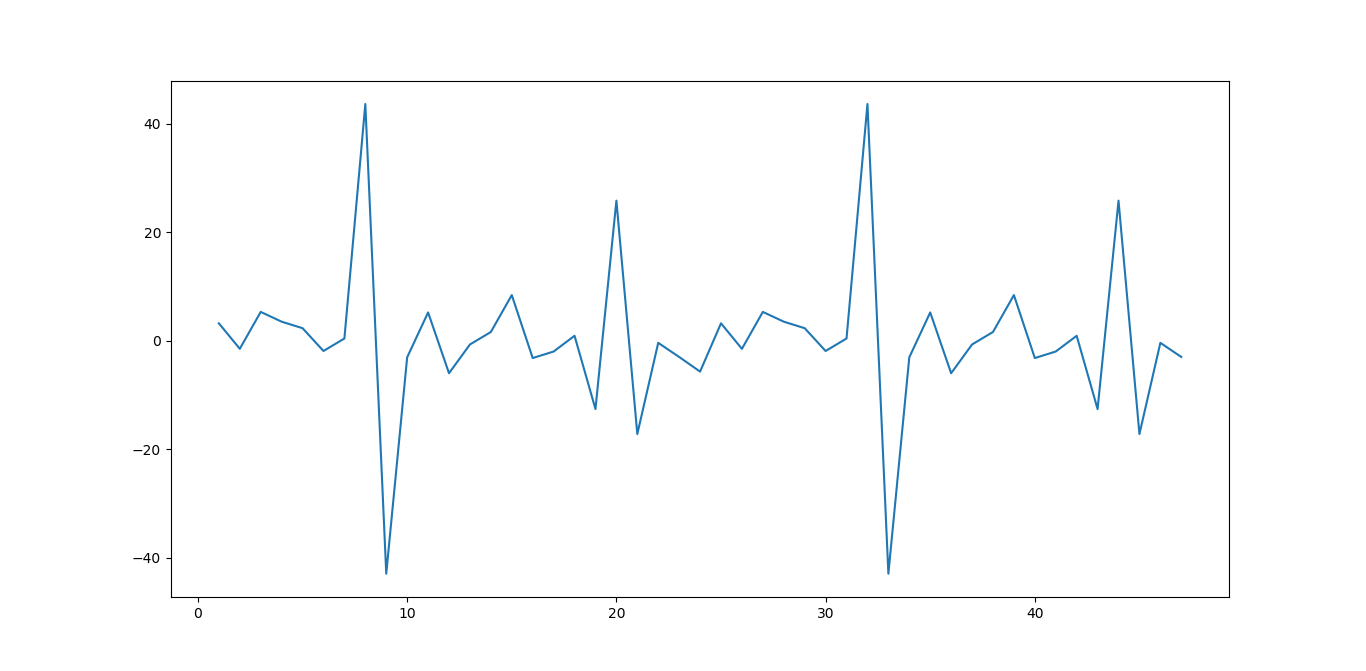从图上看，一阶差分后，感觉数据分布更对称为随机了。
#自相关图
plot_acf(D_data).show()
plt.show()

结果如下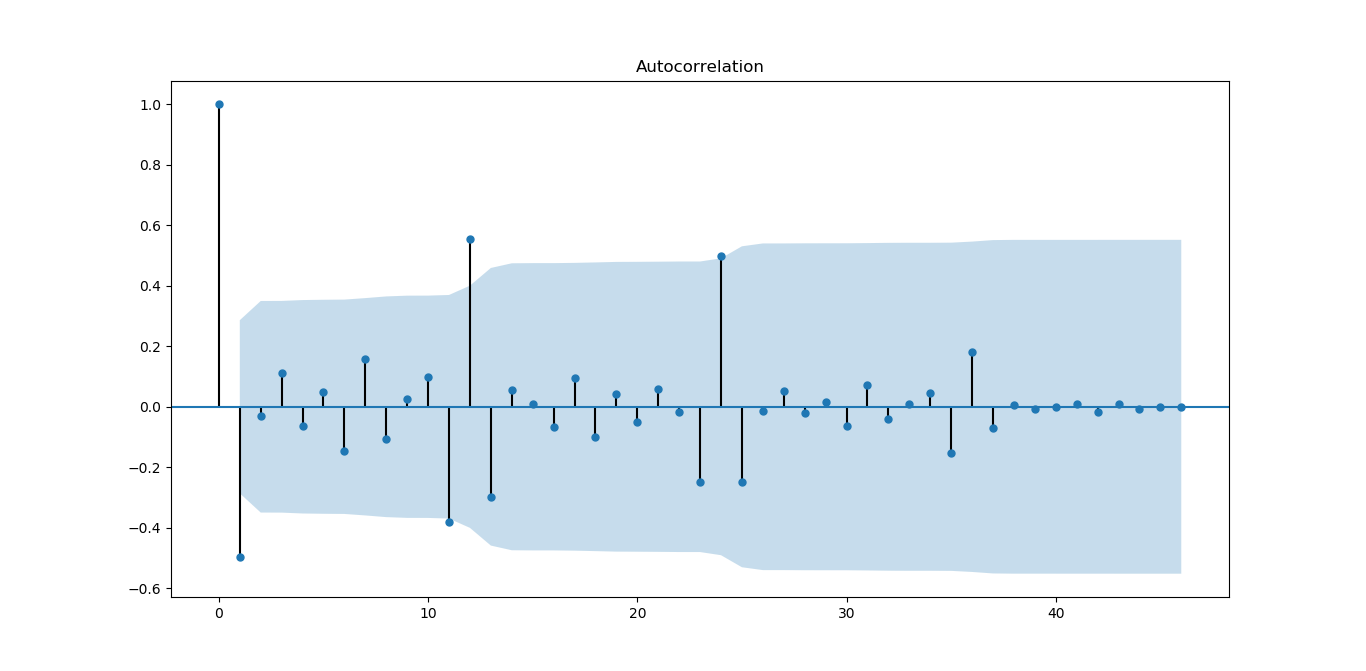从图上看，一阶差分对数据有改善，振荡衰减更明显
#偏自相关图
plot_pacf(D_data).show()

结果如下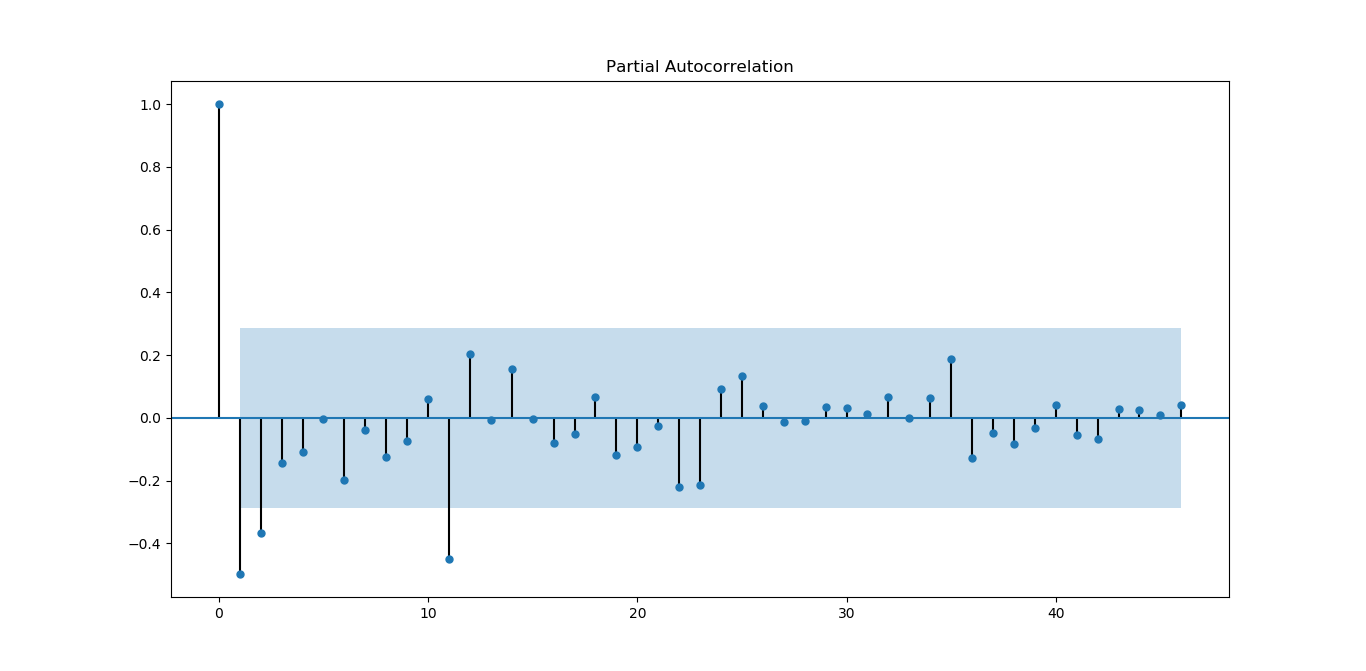从图上看，偏相关系数虽然没有自相关系数好，但基本能满足平稳序列的要求。
#平稳性检测
print('差分序列的ADF检验结果为：')
print( ADF(D_data))

结果如下
差分序列的ADF检验结果为：
(-4.595500765524432, 0.0001316587309452837, 10L, 36L, {'5%': -2.9459512825788754, '1%': -3.626651907578875, '10%': -2.6116707716049383}, 270.35268975914374)

p值远小于0.05，拒绝原假设（原假设认为存在单位根），所以该序列为平衡时间序列。
#白噪声检验
from statsmodels.stats.diagnostic import acorr_ljungbox
#返回统计量和p值
print('差分序列的白噪声检验结果为：')
print(acorr_ljungbox(D_data, lags=1))

结果如下：
差分序列的白噪声检验结果为：
(array([12.35619393]), array([0.00043953]))

P=0.00043953，统计量的P值小于显著性水平0.05，则拒绝原假设（原假设认为为白噪声序列），所以一阶差分后该序列为非白噪声序列，有进一步分析的价值。
Holt-Winters模型原理及python实现
本部分来源，也可以参考该文
原理分析
公式前面也写过，这里再表述一下，有时间再来整理
假设随机时间序列为：
$x_t(t=1,2,\ldots,N,N=n)$
时间序列数据一般有以下几种特点：1.趋势(Trend)  2. 季节性(Seasonality)。
趋势描述的是时间序列的整体走势，比如总体上升或者总体下降。下图所示的时间序列是总体上升的：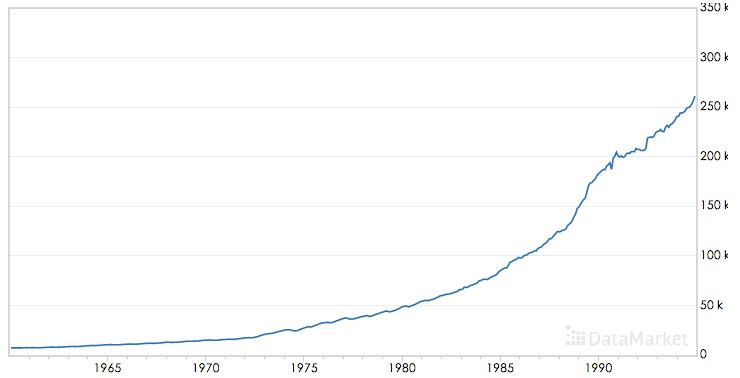季节性描述的是数据的周期性波动，比如以年或者周为周期，如下图：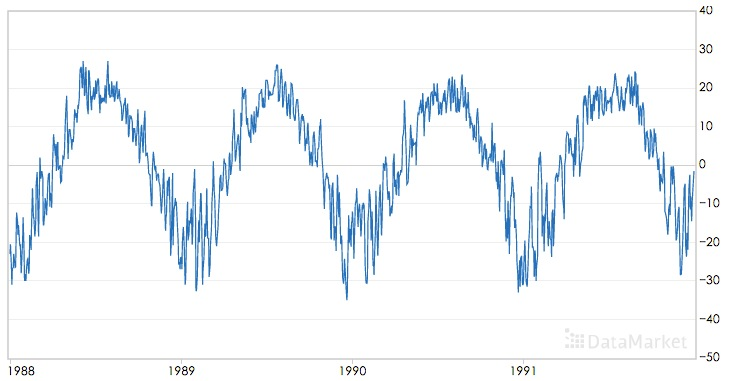三次指数平滑算法可以对同时含有趋势和季节性的时间序列进行预测，该算法是基于一次指数平滑和二次指数平滑算法的。
移动平均(The simple moving average (MA))
直观上,最简单的平滑时间序列的方法是实现一个无权重的移动平均,目前已知的方法是用窗口函数,平滑统计量 $S_t$就是最近k个观察值的均值。公式如下:
$s_t=\frac{1}{k} \sum_{n=0}^{k-1} x_{t-n}=\frac{x_t+x_{t-1}+\ldots + x_{t-k+1}}{k}=s_{t-1}+\frac{x_t -x_{t-k}}{k}$
这样的方法存在明显的缺陷,当k比较小时,预测的数据平滑效果不明显,而且突出反映了数据最近的变化;当k较大时,虽然有较好的平滑效果,但是预测的数据存在延迟。而且最少需要k个值(窗口有限)。
加权移动平均
一种稍微复杂的方法是先选择一组权重因子来计算加权移动平均
${\omega_1,\omega_2,\ldots,\omega_k},满足\sum_{n=1}^{k}\omega_n=1$
然后用这些权重来计算这些平滑统计量:
$s_t=\sum_{n=1}^{k}\omega_n x_{t-n+1}=\omega_1x_t+\omega_2 x_{t-1}+\ldots+\omega_{k}x_{t-k+1}$
在实践中,通常在选择权重因子时,赋予时间序列中的最新数据更大的权重,并减少对旧数据的权重。这个方法也需要最少k个值,并且计算复杂。
简单指数平滑法
幸运地是有一种方法可以避免上述问题,它叫做指数平滑法。最简单的指数平滑法如下:
$s_i = \alpha x_i + (1-\alpha)s_{i-1}$
其中$\alpha$是平滑因子,$0 < \alpha < 1$。$s_i$是当前时刻的平滑值。换句话说,平滑统计值$S_i$是当前统计值$X_t$与上一时间平滑值$S_{i-1}$加权平均。这个简单指数平滑是很容易被应用的,因为只要有两个观察值就能计算了。这里α的选取,我们可以采用最小二乘来决定$\alpha$(最小化$(s_t-x_t)^2$)。
一次指数平滑算法进行预测的公式为：
$x_{i+h}=s_i$
其中i为当前最后的一个数据记录的坐标，亦即预测的时间序列为一条直线，不能反映时间序列的趋势和季节性。
为什么被称为“指数”平滑法
从它的递推公式就能发现:
简单指数平滑法适用于没有总体趋势的时间序列。如果用来处理有总体趋势的序列,平滑值将往往滞后于原始数据,除非α的值接近1,但这样一来就会造成不够平滑。
二次指数平滑（HoltWinters-无季节趋势）
为了解决上述问题,于是引出了二次指数平滑,能够保留总体趋势信息。因为将指数平滑应用了两次,所以被称为二次指数平滑。与简单指数平滑相比,二次指数平滑加入了时间趋势统计量$t_i$,公式如下:
$s_i=\alpha x_i + (1-\alpha)(s_{i-1}+t_{i-1})\\ t_i=\beta (s_i-s_{i-1}) + (1-\beta)t_{i-1}$
$s_i$添加了与一次指数平滑相比，添加了一个新的趋势项$t_i$；而$t_i$的表达式与一次指数平滑形式上是一样的，只不过用将$x_i$换成$(s_i-s_{i-1})$,趋势可以想像成增量，就是在此刻与上一刻的差值，趋势当然应该用平滑后的数据的差值；同时将一次平滑中的$s_{i-1}$换成$t_{i-1}$
二次指数平滑的预测公式为
$x_{i+h}=s_i+h \ t_i$
二次指数平滑的预测结果是一条斜的直线
三次指数平滑
三次指数平滑将时间序列的季节性这一特征也考虑进去了。
季节性被定义为时间序列数据的趋势,它表现出每一个周期重复自身的行为,就像任何周期函数一样。“季节”这个词用来表示行为每隔时间段L就开始自我重复。在自然界中有不同类型的季节性“累加性”(additive)和“累乘性“(multiplicative),就像加法和乘法是数学的基本运算。
如果每个12月都比每个11月多卖出1000套公寓,我们就说这样的季节趋势是“累加性”的。可以用绝对增⻓来表示。如果我们在夏季比冬季多卖出10%的公寓,那么季节趋势在自然中是“累乘性”的。
累乘性公式如下:
下面的式子中$k$表示季节频率，这个还需要查资料进行理解
$s_i=\alpha \frac{x_i }{p_{i-k}} +(1-\alpha)(s_{i-1}+t_{i-1})\\ t_i=\beta (s_i-s_{i-1}) + (1-\beta)t_{i-1}\\ p_i=\gamma \frac{x_i}{s_i} + (1-\gamma)p_{t-k}\\$
上式中k为周期， 累乘三次指数平滑的预测公式为：
$x_{i+h}=(s_i+h\ t_i)p_i-k+(h\ mod\ k)$
或者
$x_{i+h}=(s_i+h\ t_i)p_{i-k+1+(h-1) \ mod \ k}$
两个公式还需要确认下
累乘性公式初始值的计算
$s_k=\frac{1}{k} (s_1+s_2+\cdots+s_k)\\ t_k=\frac{1}{k}\left[ \frac{x_{k+1}-x_1}{k}+\frac{x_{k+2}-x_2}{k}+\cdots+\frac{x_{k+k}-x_k}{k} \right]\\ p_1=\frac{x_1}{s_k},p_2=\frac{x_2}{s_k},\cdots,p_k=\frac{x_k}{s_k}$
累加性公式如下:
$s_i=\alpha (x_i-p_{i-k} )+(1-\alpha)(s_{i-1}+t_{i-1})\\ t_i=\beta (s_i-s_{i-1}) + (1-\beta)t_{i-1}\\ p_i=\gamma (x_i-s_i) + (1-\gamma)p_{i-k}\\ x_{i+h}=s_i+hb_i + p_{i-k+h \ mod \ k}$
或者
$x_{i+h}=s_i+hb_i + p_{i-k+1+(h-1) \ mod \ k}$
两个公式还需要确认下
累加性公式初始值的计算
$s_k=\frac{1}{k} (s_1+s_2+\cdots+s_k)\\ t_k=\frac{1}{k}\left[ \frac{x_{k+1}-x_1}{k}+\frac{x_{k+2}-x_2}{k}+\cdots+\frac{x_{k+k}-x_k}{k} \right]\\ p_1=x_1-s_k,p_2=x_2-s_k,\cdots,p_k=x_k-s_k$
其中 $\alpha$是数据平滑因子, $0 < \alpha < 1$;$\beta$是趋势平滑因子,$0 < \beta < 1$; $\gamma$是季节改变平滑因子$0 < \gamma < 1$。
$\alpha，\beta，\gamma$的值都位于[0,1]之间，可以多试验几次以达到最佳效果。
$s,t,p$初始值的选取对于算法整体的影响不是特别大，通常的取值为$s_0=x_0,t_0=x_1-x_0$,累加时$p=0$,累乘时$p=1$.
对三次指数平滑法而言,我们必须初始化一个完整的“季节”$p_i$的值,不过我们可以简单地设置为全1(针对累乘式)或全0(针对累加式)。只有当序列的⻓度较短时,我们才需要慎重考虑初始值的选取。
我们这里讲的Holt-Winters模型就是三次指数平滑法。哇,终于切入正题了。
所有的指数平滑法都要更新上一时间步⻓的计算结果,并使用当前时间步⻓的数据中包含的新信息。它们通过“混合”新信息和旧信息来实现,而相关的新旧信息的权重由一个可调整的拌和参数来控制。各种方法的不同之处在于它们跟踪的量的个数和对应的拌和参数的个数。三次指数平滑法,功能最强大,既能体现趋势性又能体现季节性,所以三次指数平滑法的参数最多,有三个。
下图为使用累加三次指数平滑进行预测的效果：其中红色为源时间序列，蓝色为预测的时间序列，$\alpha，\beta，\gamma$的取值为0.45，0.2，0.95：下图为累乘三次指数平滑进行预测的效果，$\alpha，\beta，\gamma$的取值为0.4，0.05，0.9：可以看到三次指数平滑算法可以很好的保存时间序列数据的趋势和季节性信息，在International Airline Passengers数据集上累乘平滑指数算法的效果更好。
python代码实现
我们知道HoltWinters模型有三个可调参数,我们的目的就是训练出有效的α,β, γ
。我们有两种方法,一种就是自己取值来试试,一种就是采用数值优化的思想,比如
前面我们提到的最小二乘来最小化误差来求参数(注意不一定能全局收敛!这个问题
实在是让人头痛。。。)我们就采用最小二乘法(L-BFGS)。
RMSE的实现
statsmodels中的holtwinters
statsmodels.tsa模块
statsmodels.tsa.holtwinters源码
statsmodels.tsa.holtwinters.ExponentialSmoothing
ExponentialSmoothing - 指数平滑算法
基于SVM作短期时间序列的预测
传统的做法是提取1、2、3、4、5、7、9、13个单位时间的数据作为特征进行预测；
举个例子进行分析，比如每天都有口香糖的销量，那么如何通过几周的数据预测明天的数据，
就可以选择前1、2、3、4、5、7、14天的数据作为特征，从而预测明天的数据，
通过构建特征，再选择核函数进行预测，其中调参的参数尽量要进行最优化，
参考方法：如果选择RBF核函数，那么其中就会有三个参数，固定两个，然后不停的优化另外一个，直到得到最优解。
具体应用的例子：
（1）SVM预测风场：http://wenku.baidu.com/link?url=SCCIJJe8tXLbTjLMZ81x5Qy6elsceAKIOwtkZ0QxfSCQQ4KaWKwo8Biepjs3Ss2LJ2ewhisNR0ixrDY4kV1Rd7BcqWRenuTaG85K80E-30y
（2）SVM预测股票指数：基于SVM修正的模糊时间序列模型在沪指预测中的应用
（3）SVM预测时间序列其他方面：http://www.docin.com/p-233353900.html
LSTM模型分析及对时序数据预测的具体实现（python实现）
来源：2017年09月30日 10:28:08
如何用LSTM自编码器进行极端事件预测？(含Python代码)
时间序列的并行实现
spark里面的库是没有时间序列算法的，但是国外有人已经写好了相应的算法。其github网址是:https://github.com/sryza/spark-timeseries
sryza/spark-timeseries
Spark-TimeSeries使用方法
A New Library for Analyzing Time-Series Data with Apache Spark
【Spark Summit East 2017】使用Spark进行时间序列分析
基于小波变换的时间序列预测，Python实现，来自雪球
清华AIOps算法：KPI聚类
其他资料
时间序列挖掘-预测算法-三次指数平滑法(Holt-Winters)
Holt-Winters原理和初始值的确定
时间序列模型Prophet使用详细讲解


展开全文• 测试序列稳定：看以看到整体的序列并没有到达稳定要求，要将时间序列转为平稳序列，有如下几种方法：DeflationbyCPILogarithmic（取对数）FirstDifference（一阶差分）SeasonalDifference（季节差分）...
• data = pd.read_excel('时间序列预测数据集.xlsx') # data.columns=[时间,投递人数,投递次数,工程师投递人数,工程师投递次数,招聘发布公司量,发布职位量,工程师岗位发布公司,工程师岗位发布量] for i in dat
import numpy as np
import pandas as pd
import matplotlib.pyplot as plt
from statsmodels.tsa.api import ExponentialSmoothing

data = pd.read_excel('时间序列预测数据集.xlsx')
# data.columns=[时间,投递人数,投递次数,工程师投递人数,工程师投递次数,招聘发布公司量,发布职位量,工程师岗位发布公司,工程师岗位发布量]

for i in data.drop('时间',axis=1).columns:
y = data[i][:37]
ets = ExponentialSmoothing(y, trend='add', seasonal='add', seasonal_periods=12)
r = ets.fit()
pred = r.predict(start=len(y), end=len(y) + 12)
data[i][37:] = pred
pd.DataFrame({
'origin': data[i],
'fitted': r.fittedvalues,
'pred': pred
}).plot(legend=True)
plt.title(i)
data


https://www.cnblogs.com/lfri/p/12243268.html


展开全文• 时间序列可以分为长期趋势（trend）、季节变动（seasonal）、循环变动（cycling）和随机波动（irregular）四个部分。 长期趋势（ T ）：在较长时期内受某种根本因素作用而形成的总的变动趋势 季节变动（ S ）：在...数据分析 机器学习
• 一个季节性时间序列中会包含三部分，趋势部分、季节性部分和无规则部分。分解时间序列就是要把时间序列分解成这三部分，然后进行估计。 对于可以使用相加模型进行描述的时间序列中的趋势部分和季节性部分，...R
• 白噪声模型 时间序列算法之ARIMA模型 对非平稳时间序列的分析方法可以分为确定因素分解的时序分析和随机时序分析两个...根据时间序列的不同特点，随机时序分析可以建立的模型有ARIMA模型、残差自回归模型季节模型.
• 1 时间序列预测模型 1.1 时间序列分解 时间序列是指将同一 统计量的数值按其发生的时间先后顺序排列而成的数列，常用按时间顺序排列的一组随机变量????1,????2,⋯????????,⋯表示 一个随机事件的时间序列，简记为 {?...
• 要本着应用到实际工作中目的去学时间序列...季节性时间序列季节性时间序列 时间序列包含什么 趋势部分 不规则部分 季节性部分 非季节性时间序列 √ √ 不包含 季节性时间序列 √ √ √ 特别强调：这里的python
• Task03：时间序列模型 时间序列分解 时间序列变化的影响： 1.长期趋势（trend）:会导致序列出现明显的长期趋势。 2.循环波动（circle）:会导致序列呈现出周期性波动。 3.季节性波动（season）：会导致序列呈现出和...
• 时间序列预测 自相关与偏自相关 ...稳定时间序列 ARIMA模型 ...在需要考虑季节性上的模型使用SARIMA模型 SARIMA模型 Jupyter 设置 In : %%javascript IPython.OutputArea.
• 本文从比利时皇家天文台的太阳黑子指数数据中心网站获得1700年至2016年的太阳黑子年度数据，时间跨度为317年，共获得317个数据，对数进行分析，建立季节时间序列模型，并对模型进行检验，最后对太阳黑子数据进行预测...
• 目录1 概述2 时间序列中的季节成分3 机器学习的好处4 季节性的类型4 消除季节性5 每日最低温度数据6 差分6.1 每日数据的差分6.2 月平均数据的差分7 通过建模来进行修正 1 概述 时间序列数据可能包含一些季节性的...python 机器学习
• 本次，开始分解季节性时间序列。 一个季节性时间序列中会包含三部分，趋势部分、季节性部分和无规则部分。分解时间序列就是要把时间序列分解成这三部分，然后进行估计。 对于可以使用相加模型进行描述的时间...
• 模糊时间序列模型季节模型都是基于时间序列的模型，为了探讨在时间序列表现出一定的周期时，哪种模型的预测效果会更好，分别利用模糊时间序列模型季节模型对南京某商场的客流量进行预测，计算并比较两种方法下...
• 并应用熵权法确定各参数权重，建立工作面涌水量预测的非线性回归修正模型，并将模拟预测结果与忽略季节效应的ARIMA模型预测的涌水量进行对比，结果表明，建立的非线性时间序列模型计算的涌水量更为接近实测涌水量，...
• 时间序列数据集可以包含季节性成分。这是一个随时间重复的周期，如每月或每年。这种重复的循环可能会模糊我们在预测时希望建模的信号，从而可能为我们的预测模型提供一个强大的信号。 可以看出有很强的季节性成分...
• 时间序列可以分为长期趋势（trend）、季节变动（seasonal）、循环变动（cycling）和随机波动（irregular）四个部分。 长期趋势（ T ）现象在较长时期内受某种根本因素作用而形成的总的变动趋势 季节变动（ S ）...
• 建模与分析方法：时间序列模型，利用差分自回归移动平均模型 (Autoregressive Integrated Moving Average Model, 简记 ARIMA) 进行分析预测。 参考资料： ...
• 主要运用时间序列分析的方法及EVicws与SPSS统计软件对时间序列建立乘积季节模型，并搜集了中国1993年1月～2000年12月的民航货运量数据，对其进行分析。根据Box-Jenkins的建模方法，建立了中国民航货运量的模型，并对...
• 季节性自回归综合移动平均（SARIMA）或季节性ARIMA是ARIMA的一个扩展，它明确支持具有季节性分量的单变量时间序列数据，它增加了三个新的超参数来指定序列季节性成分的自回归（AR）、差分（I）和移动平均（MA），...
• 本文聊聊arima模型。ARIMA是一种比较常用的基于历史数据来做预测的时间序列模型。时间序列在商业中有很多应用，比如它可以解释销售中的季节性规律；可以预测新客或流失客户......